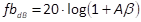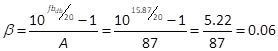Previously, we determined that the gain of the input stage is 74.6, or equivalently 37.45 dB. We also discussed that the peak amplitude of the input signal that can be given to the power stage is 12V, and that the concertina phase splitter practically forwards, to the power stage, almost the entire output signal produced by the input stage.

Under these conditions, the input stage produces a 12V peak amplitude output signal when the peak amplitude of the input signal is just Vin=12V/74.6=0.16V. Generally, input sources produce a much larger signal. As we already said, some input sources, as for instance CD players or DACs, can even provide a 2.5V or 3V peak signal.

For instance, if we want to produce the maximum output signal, with a 2.5V peak signal, the gain needed at the input stage is just A=12V/2.5V=4.8, or equivalently 13.62 dB. Accordingly, we can apply a global negative feedback up to fbdb=37.45-13.62=23.83 dB and we are still able to have the maximum output power.

If we want to obtain maximum output power also with sources providing less input voltage, as for instance just 1V peak, the needed gain of the input stage is A=12V/1V=4.8 or equivalently 21.58 dB.   This gives space for a negative feedback fbdb=37.45-21.58 =15.87 dB.

The amount of negative feedback is determined by the feedback factor β, as discussed in Section 4.4. The value of β can be computed knowing the gain of the amplifier, when no negative feedback is used. This, in turn, can be determined using the gain of the individual stages, which we have computed in previous sections. The voltage gain of the amplifier with no negative feedback is A=74.6∙0.98∙1.19=87, or alternatively Adb=37.45-0.18+1.51=38.78 dB.

As discussed in Section 4.4.1, the amount of feedback is. When we apply a feedback fbdb=15.87 dB, to provide maximum power with 1V peak input signal with an amplifier gain  A=87, we have that the feedback factor β is.

To inject the negative feedback, at the cathode of the input stage vacuum tube, we split the cathode resistor, used for cathode biasing, into two resistors Rk and R2. The negative feedback is injected between the two resistors through the resistor R1, as depicted in Figure 52. Remember that. Therefore, if resistor R2 is set to 150 Ohm, β=0.06 can be approximatively obtained by choosing the feedback resistor R1=2.2K Ohm. The cathode resistor Rk, which was previously set to 870 Ohm, should now be reduced, to take into account the 150 Ohm used for R2. The cathode resistor should be 870 Ohm–150 Ohm=720 Ohm. The closest standard is 680 Ohm, which is near to the computed value.